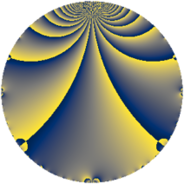# Properties

 Label 23.2.cLevel $23$ Weight $2$ Character orbit 23.c Rep. character $\chi_{23}(2,\cdot)$ Character field $\Q(\zeta_{11})$ Dimension $10$ Newform subspaces $1$ Sturm bound $4$ Trace bound $0$

# Related objects

## Defining parameters

 Level: $$N$$ $$=$$ $$23$$ Weight: $$k$$ $$=$$ $$2$$ Character orbit: $$[\chi]$$ $$=$$ 23.c (of order $$11$$ and degree $$10$$) Character conductor: $$\operatorname{cond}(\chi)$$ $$=$$ $$23$$ Character field: $$\Q(\zeta_{11})$$ Newform subspaces: $$1$$ Sturm bound: $$4$$ Trace bound: $$0$$

## Dimensions

The following table gives the dimensions of various subspaces of $$M_{2}(23, [\chi])$$.

Total New Old
Modular forms 30 30 0
Cusp forms 10 10 0
Eisenstein series 20 20 0

## Trace form

 $$10 q - 7 q^{2} - 7 q^{3} - 3 q^{4} - 3 q^{5} + 6 q^{6} - 5 q^{7} + 4 q^{8} - 2 q^{9} + O(q^{10})$$ $$10 q - 7 q^{2} - 7 q^{3} - 3 q^{4} - 3 q^{5} + 6 q^{6} - 5 q^{7} + 4 q^{8} - 2 q^{9} + q^{10} + 7 q^{11} + 12 q^{12} - 3 q^{13} + 9 q^{14} + 12 q^{15} + q^{16} - 10 q^{17} - 14 q^{18} + 2 q^{19} - 9 q^{20} - 2 q^{21} - 6 q^{22} - 12 q^{23} - 38 q^{24} - 4 q^{25} + 12 q^{26} - 4 q^{27} + 7 q^{28} + 14 q^{29} + 7 q^{30} + 10 q^{31} + 21 q^{32} + 16 q^{33} + 29 q^{34} + 7 q^{35} + 27 q^{36} - 19 q^{37} - 8 q^{38} + q^{39} + q^{40} + 7 q^{41} - 25 q^{42} - 11 q^{43} - 34 q^{44} - 6 q^{45} - 29 q^{46} - 18 q^{47} + 18 q^{48} - 18 q^{49} + 16 q^{50} + 7 q^{51} - 20 q^{52} + 29 q^{53} - 6 q^{54} - q^{55} - 2 q^{56} - 8 q^{57} - 23 q^{58} - 21 q^{59} + 25 q^{60} + 3 q^{61} + 4 q^{62} + 34 q^{63} + 24 q^{64} + 2 q^{65} + 2 q^{66} + 45 q^{67} - 30 q^{68} + 26 q^{69} + 38 q^{70} - 14 q^{71} + 19 q^{72} + 19 q^{73} + 10 q^{74} - 28 q^{75} - 16 q^{76} + 2 q^{77} - 4 q^{78} - 15 q^{79} - 52 q^{80} - 44 q^{81} + 16 q^{82} + 18 q^{83} - 17 q^{84} - 19 q^{85} - 11 q^{86} - 23 q^{87} + 27 q^{88} + 25 q^{89} - 20 q^{90} - 4 q^{91} + 52 q^{92} + 4 q^{93} + 17 q^{94} + 6 q^{95} - 51 q^{96} - 34 q^{97} + 17 q^{98} - 30 q^{99} + O(q^{100})$$

## Decomposition of $$S_{2}^{\mathrm{new}}(23, [\chi])$$ into newform subspaces

Label Dim $A$ Field CM Traces $q$-expansion
$a_{2}$ $a_{3}$ $a_{5}$ $a_{7}$
23.2.c.a $10$ $0.184$ $$\Q(\zeta_{22})$$ None $$-7$$ $$-7$$ $$-3$$ $$-5$$ $$q+(-\zeta_{22}+\zeta_{22}^{4}-\zeta_{22}^{5}+\zeta_{22}^{6}+\cdots)q^{2}+\cdots$$